TLDR; DAX is a powerful query language used in tools such as Power BI. In this post, we explain how to better understand the results obtained through DAX formulas by being aware of the different types of Evaluation Contexts and how these affect our code.

One of the most important features of the DAX language is the notion of Evaluation Contexts. The evaluation context is the context under which the formulas we write in DAX will be applied.

## What is Evaluation Context and why is it important?

Let's illustrate what Evaluation Context does through an example. Say we have data on the sales of certain products from a store. The Sales has columns containing the unit Cost, the quantity of products sold, and the total amount of sales (among others). The Product table contains columns with details about the products sold, such as their colour or brand.

If we want to calculate a measure that gives the total amount of sales, we can create a new measure with the following line of DAX code:

``````TotalSales = SUM ( Sales[Sales Amount] )
``````

Only very little DAX-specific knowledge is needed to understand what this does: the function SUM aggregates values in the column SalesAmount in the Sales table and sums them.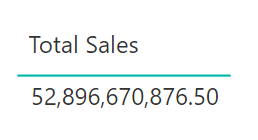Note that I didn't write “all values in the column SalesAmount”, but simply “values”. This was deliberate, as simply looking at this line of DAX code doesn't provide us with enough information to know which cells will be aggregated before computing the sum.

This is because we don't know what the Evaluation Context is here.

The evaluation context is everything around the formula that will constrain the values over which our formula is applied. This is why our formula can be the same and yet give very different results depending on the Evaluation Context.

By adding a slicer in the page to filter by colour, for example, the result of our Total Sales measure will change.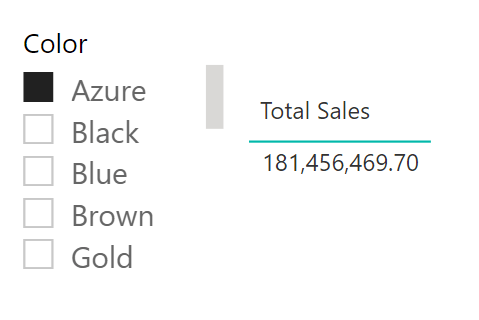It is always important to remember that the value of a formula depends on its context.

## Two types of Evaluation Contexts

### Filter Context

The first kind of evaluation that DAX uses is the filter context. These are all the filters applied to your data before executing any DAX command. In the example above, the filter Color: Azure is the filter context.

It is important to note that the source of the filter context will depend on the tool you are using. For instance, selecting a data point in a chart will automatically filter the whole page by that value in Power BI, however this is not the case in Excel spreadsheets.

The filter context can be defined by:

• Row Selection
• Column Selection
• Report Filters
• Slicers Selection

### Row Context

The second kind of evaluation that DAX uses is the row context. In certain scenarios, a DAX query might need to evaluate every row in a table one at a time, iterating through and performing a calculation on each row. This row context can't be set by the user, it is activated by the specifics of the DAX query being executed. Some common DAX functions like SUM, AVERAGE and MIN have equivalent aggregators that work in this iterative, row by row context. They're commonly known as X aggregators, as the function names have an X appended to them - e.g. SUMX, AVERAGEX and MINX.

In our first example, we used the Sales Amount column in the Sales table to calculate the Total Sales measure. Now imagine the Sales Amount column doesn't exist in our table, and we want to calculate it by multiplying the Unit Price by the Quantity. How do we multiply a column by another column? We iterate through each row and multiply Unit Price by Quantity in each row.

Let's create a calculated column with the following expression:

`Sales Amount = Sales[Unit Price] * Sales[Quantity]`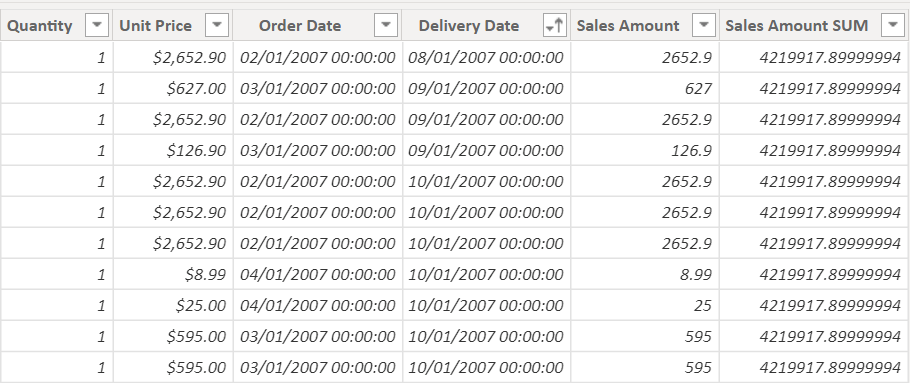In this table, Sales Amount is calculated by iterating through the rows one by one and applying the formulas for Sales Amount in that row.

The following line of code does something similar:

`Sales Amount = SUMX ( Sales, Sales[Unit Price] * Sales[Quantity] )`

SUMX will iterate through the rows one by one and apply the formula in that row. Only at the end will it add all of these values together, which is the result of Sales Amount SUM.

The row context is what specifies for which row we are multiplying the values. So whenever there is the notion of a “current row”, a row context is being set.

## CALCULATE Function

The `CALCULATE` function is the only function in DAX that can change and update the filter context.

`CACLULATE` works with a very simple syntax. You simply pass as arguments the expression you want to calculate followed by the set of filters you want to apply to the data before calculating the expression.

``````CALCULATE (
Expression,
Filter 1,
…,
Filter n
)
``````

First, the filters are applied to the evaluation context, and then the expression is evaluated. This is very powerful, as you have the ability to change the evaluation context regardless of what the original evaluation context was.

The following formula always computes the sales for blue products.

`Blue Sales CALCULATE = CALCULATE ( [Total Sales], 'Product'[Color] = "Blue" )`

Here, we are first filtering all the products of our table by the colour blue, and then calculating the sum of the TotalSales. We now know the exact context in which we are calculating the SUM, because CALCULATE applies the filters we specify on the whole table, it doesn't add them to other evaluation filters.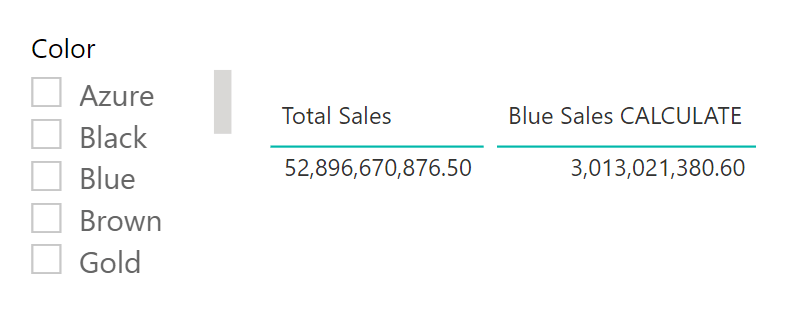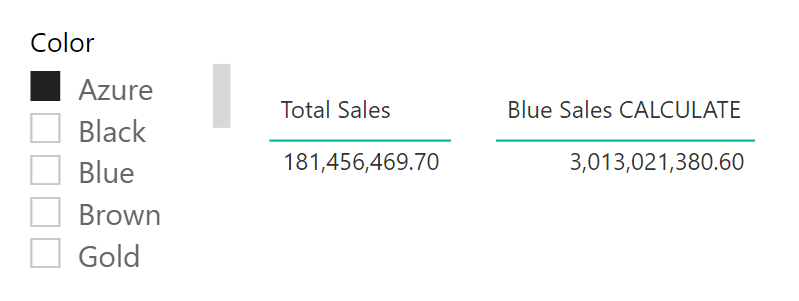We can see how applying a filter for the colour Azure changes the Total Sales measure but not our `Blue Sales CALCULATE` measure.

## FILTER and ALL Functions

You might be wondering why other functions, such as `FILTER` or `ALL` can't also change the filter context if, after all, they are also filtering out or removing filters from the data.

### FILTER

`FILTER` does not change the filter context, because it doesn't first apply a filter context and then calculate the measure over it. The calculation happens as a result of table calculations, meaning the function will iterate through the rows of the table and execute the measure applying the specified filter.

``````Sum of Black Sales =
SUMX (
FILTER (
'Sales',
'Sales'[Quantity] > 1
),
Sales[Unit Cost] * Sales[Quantity]
)
``````

In this example, SUMX will iterate through the rows of the Sales table in the current filter context, and calculate `Sales[Unit Cost] * Sales[Quantity]` for the rows where the quantity is greater than 1. So the filter context is never modified.

### ALL

The same applies for the function `ALL`, which ignores the filter context in a table or a column. In the following example, `ALL(Sales)` returns the table Sales without a filter context. Then, `SUMX` computes the sum of all the values in the Sales Amount column of the Sales table.

`Sales Amount All = SUMX ( ALL (Sales), Sales[Sales Amount] )`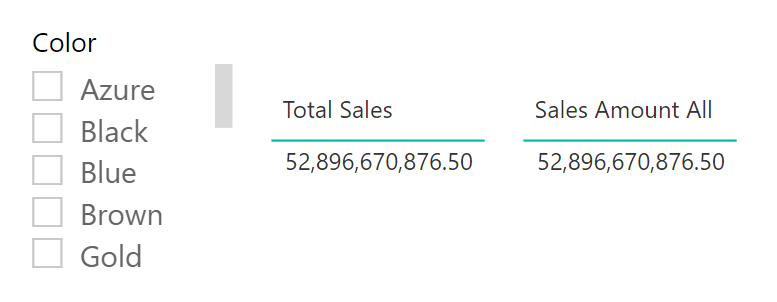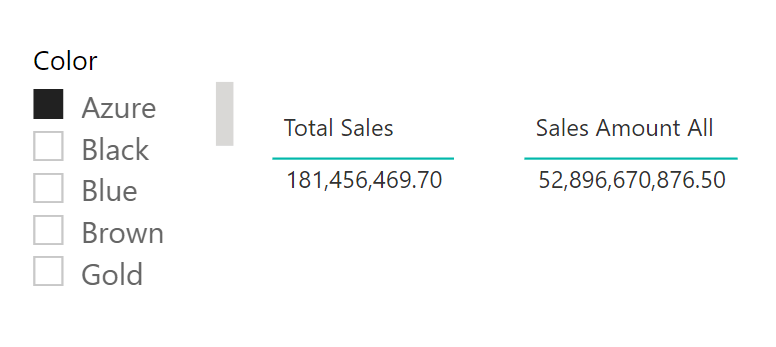Now, filtering by a colour in the slicer doesn't affect the Sales Amount All measure.

## Conclusion:

We have seen how evaluation contexts affect our DAX code. Understanding that the evaluation context is made up of a filter context and a row context, when each takes effect, and how they are defined allows us to better understand our results and avoid mistakes. Remember: the value of a formula depends on its context. So, if your formula is correct but you are not getting the result you expect, you might be forgetting about the evaluation context!

### Elisenda Gascon

#### Apprentice Engineer IIElisenda was an Apprentice Engineer from 2021 to 2023 at endjin after graduating with a mathematics degree from UCL. Her passion for problem solving drove her to pursue a career in software engineering.

During her time at endjin, she helped clients by delivering data analytics solutions, using tools such as Azure Synapse, Databricks notebooks, and Power BI. Through this, she also gained experience as a consultant by delivering presentations, running customer workshops, and managing stakeholders.

Through her contributions to internal projects, she gaines experience in web development in ASP.NET, contributed to the documentation of the Z3.Linq library, and formed part of a team to develop a report to explore global trends in wealth & health.

During her training as a software engineer, Elisenda wrote a number of blog posts on a wide range of topics, such as DAX, debugging NuGet packages, and dependency injection. She has also become a Microsoft certified Power BI analyst and obtained the Green Software for Practitioners certification from the Linux Foundation.